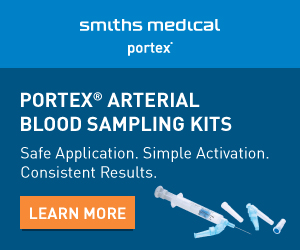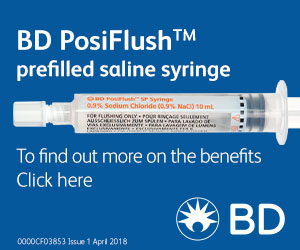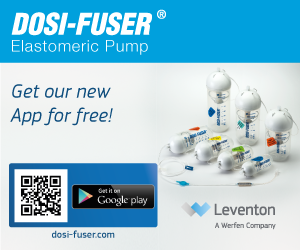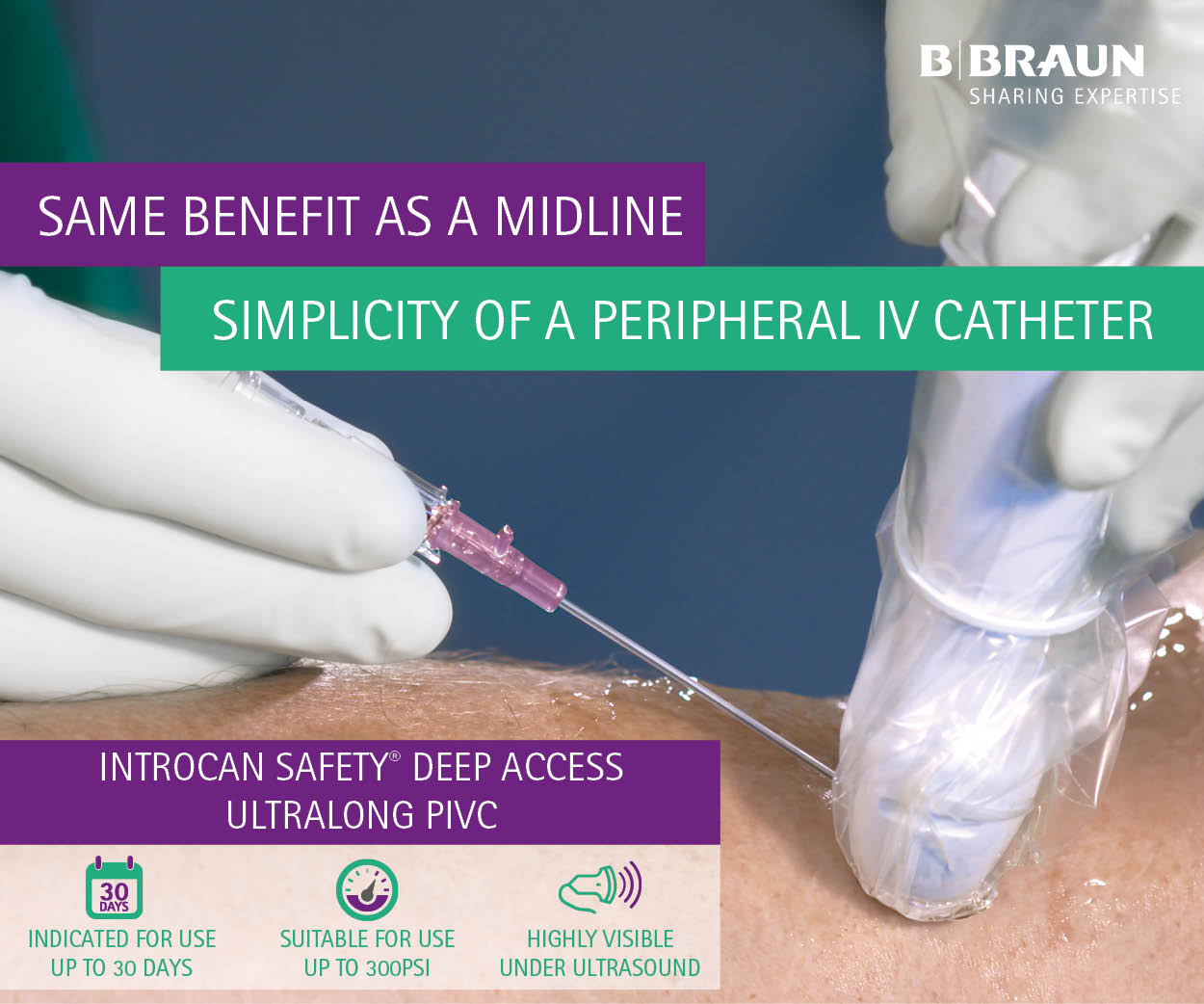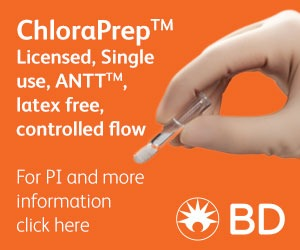# Measurement of osmolality of parenteral nutrition solutionsAbstract:

BACKGROUND: our aim was to measure the osmolality of several PN formulas at different component concentrations to determine if equations described in literature to calculate osmolarity accurately predict osmolalality in other experimental conditions different than these used to develop them.

METHODS: osmolality of 12 different types of PN solutions, 9 for central and 3 for peripheral perfusion were measured by using freezing point depression in cross-sectional study. We evaluated the agreement (Pearson correlation test) and differential bias between measured osmolality and calculated osmolarity for three different equations described in the literature: Pereira Da Silva, ASPEN Practice Manual and ASPEN guidelines.

RESULTS: mean ± SD osmolality of PN solutions was 1789 ± 256 (range 1 540 – 2 372) and 751 ± 64 mOsm/kg (range 689 – 817) for central and peripheral infusion, respectively. The osmolality of PN formulations was mainly due to glucose (r = 0.975) and amino acids (r = 0.948). All studied equations had a good correlation in the bivariate analysis (p = 0.000). All equations had a trend to underestimate the osmolality compared with the measured value. However, ASPEN guidelines equation overestimated the osmolality for peripheral PN.

CONCLUSIONS: measurement of osmolality of peripheral PN solutions is important to reduce the risk of phlebitis. The different equations described previously show a good correlation between them although in general underestimate the osmolality.

Reference:

Valero Zanuy, M.A., Pablos Bravo, S., Lazaro Cebas, A., Garcia Sanchez, J., Gomis Muñoz, P., Moreno Villares, J.M. and Leon Sanz, M. (2015) Agreement between different equations to estimate osmolarity of parenteral nutrition solutions. Nutrición Hospitalaria. 32(n06), p.2757-2762.

Thank you to our partners for supporting IVTEAM